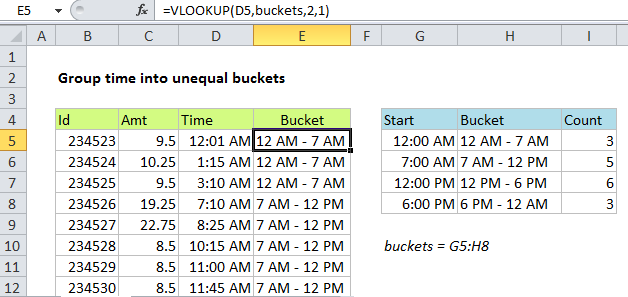# Group times into unequal buckets in Excel

This tutorial shows how to Group times into unequal buckets in Excel using the example below;

## Formula

`=VLOOKUP(time,bucket_table,column,TRUE)`## Explanation

This formula is a great example of how you can use VLOOKUP to group data in completely custom ways.

If you need to group times into buckets, but the buckets are not equal (i.e. 12 AM-7 AM, 7 AM-12 PM, etc.) you can do so with the VLOOKUP function set to approximate match.

### The problem

There are several ways to group times in Excel. If you just need to group times by the hour, a pivot table is very quick and easy. If you need to group times into other equal buckets of multiple hours (i.e. 3 hours, 4 hours, etc.) a nice solution is to use the FLOOR function. However, if you need to group times into unequal buckets, you need to take a more custom approach. VLOOKUP, in its approximate match mode, allows you to group times into custom intervals of any size.

Also See:   Excel VLOOKUP and HLOOKUP functions Examples

### The solution

The solution is to build a lookup table that “maps” each time into the right bucket. In the first column, enter the start time for the bucket. In column two, enter the name of the bucket you want to use. The table must be sorted by the start time, smallest to largest.

Also See:   Lookup with variable sheet name in Excel

Finally, configure VLOOKUP to look up each time in the bucket table with approximate match.

In the example shown, the formula n E5 is:

`=VLOOKUP(D5,buckets,2,1)`

D5 is the lookup value, “buckets” is a named range for G5:H8, 2 is the column index, and 1 is a flag that enables approximate match. (You can also use TRUE).

Also See:   How to Calculate Tax Rates in Excel

When VLOOKUP is in approximate match mode, it matches the nearest value that is less than or equal to the lookup value. In this way, you can think of the incoming lookup time as being “rounded down” into the right bucket.